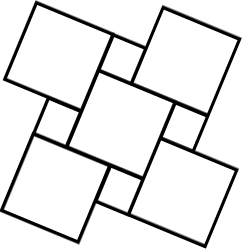# Rectangle 21663

A) Divide a rectangle measuring 126 cm x 72 cm into as many squares as possible.
b) Calculate the side length of these squares.

n =  28
x =  18 cm

### Step-by-step explanation:Did you find an error or inaccuracy? Feel free to write us. Thank you!

Tips for related online calculators
Do you want to calculate greatest common divisor two or more numbers?
Do you want to convert length units?

#### Grade of the word problem:

We encourage you to watch this tutorial video on this math problem: